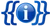Documentation

Calculates Forging stats for an item and alloy combination and outputs a table row for the forging stats template.

## Formulas

#### Item Quality

Most calculations rely on Item Quality (IQ), a hidden value that also determines the Quality category of an item.

Quality Item Quality Threshold
Craftable >= 0
Common > 1.25
Uncommon > 2.5
Rare > 4.5
Epic > 7
Legendary > 10

#### Completion Point Multiplier (CPM) and Stat Value Multiplier (SVM)

Multiplies both the amount of required Completion and the value of added stats, calculated based on Item Quality (IQ).

CPM/SVM = (5 * IQ + 10) / (IQ + 10)

Value range: 1 if IQ = 0, 5 towards IQ = Infinity

#### Impurity Limit Multiplier (ILM)

Multiplies the Impurity limit, calculated based on Item Quality (IQ).

ILM = (3 * IQ + 10) / (IQ + 10)

Value range: 1 if IQ = 0, 3 towards IQ = Infinity

#### Impurity Stat Value Multiplier (ISVM)

Additional multiplier for the value of stats, based on the Impurity Percentage (IP) of the forgeable item.

ISVM = 1 / (1 + IP * 4)

Value range: 1 if IP = 0, 0.2 if IP = 1

#### Total Completion (TC), Impurity Limit (TIL) and Stat Value (TSV)

Uses above multipliers and additional base values: Initial Value Constant (IVC), Forging Completion (FC), Max Forging Impurity (MFI) and Item Level (IL)

TC = FC * CPM

TIL = MFI * ILM

TSV = IVC * SVM * ISVM * IL

## Usage

```{{forging stats alloy row
| completion forgeable = FC - Base amount of required Completion
| completion alloy = Amount of completion provided by the alloy
| impurity forgeable = MFI - Base Impurity limit
| impurity 100 alloy = Amount of Impurity added by alloy, multiplied by 100
| alloy = Name of the alloy
| energy = Required Energy per operation
| item level = IL of the forgeable item
}}
```

## Example

 [[{{{1}}}]] Expression error: Unrecognized punctuation character "{". ItemsExpression error: Unexpected < operator. Energy Expression error: Unrecognized punctuation character "{". ItemsExpression error: Unexpected < operator. Energy Expression error: Unrecognized punctuation character "{". ItemsExpression error: Unexpected < operator. Energy Expression error: Unrecognized punctuation character "{". ItemsExpression error: Unexpected < operator. Energy Expression error: Unrecognized punctuation character "{". ItemsExpression error: Unexpected < operator. Energy Expression error: Unrecognized punctuation character "{". ItemsExpression error: Unexpected < operator. Energy
```{| class="wikitable"
{{forging stats alloy row
| completion forgeable = 1500
| completion alloy = 100
| impurity forgeable = 1500
| impurity 100 alloy = 1600
| alloy = Refined Metal Alloy
| energy = 50
| item level = 1.5
}}
|}
```